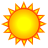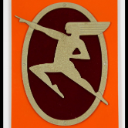# Creating a query statement

2175
2
10-17-2014 08:07 AMNew Contributor III

I would like to display a the result of a query that loops thru an array. The query statement should look the

one shown below:

countryCodes = [840, 332, 643, 724, ....];

for (var i = 0; i < countryCodes.length; i++)

{

cntryq.where =     "UN = " + countryCodes =

}

cntryq.where =     "UN = " + countryCodes =  + "OR UN = " + countryCodes =  + ....

Is this possible?

My script is attached

Tags (2)
1 Solution

Accepted SolutionsbyMVP Esteemed Contributor

You access objects in an array like

```countryCodes

```

What you should do is build a query like

"UN in (840, 332, 643, 724, ....)"

which is the same as

"UN = 840 OR UN = 332 OR ..."

So you can build the statement this way

```var inString = "";
for (var i = 0; i < countryCodes.length; i++)
{
inString += countryCodes + ", ";
}
inString.slice(0, -2);

cntryq.where = "UN in (" + inString + ")";

```
2 RepliesbyMVP Esteemed Contributor

You access objects in an array like

```countryCodes

```

What you should do is build a query like

"UN in (840, 332, 643, 724, ....)"

which is the same as

"UN = 840 OR UN = 332 OR ..."

So you can build the statement this way

```var inString = "";
for (var i = 0; i < countryCodes.length; i++)
{
inString += countryCodes + ", ";
}
inString.slice(0, -2);

cntryq.where = "UN in (" + inString + ")";

```New Contributor III

Thanks you Ken!!

Problem solved